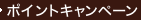•### Beginning Algebra （8 HAR/PSC）

• ただいまウェブストアではご注文を受け付けておりません。 ⇒古書を探す
• 製本 Hardcover:ハードカバー版／ページ数 589 p.
• 言語 ENG,ENG
• 商品コード 9780495118077
• DDC分類 512.9

### Table of Contents

`    Real Numbers and Their Basic Properties        1  (77)      Real Numbers and Their Graphs                2  (11)      Fractions                                    13 (15)      Exponents and Order of Operations            28 (10)      Adding and Subtracting Real Numbers          38 (9)      Multiplying and Dividing Real Numbers        47 (9)      Algebraic Expressions                        56 (7)      Properties of Real Numbers                   63 (15)        Projects                                   70 (1)        Chapter Summary                            71 (5)        Chapter 1 Test                             76 (2)    Equations and Inequalities                     78 (71)      Solving Basic Equations                      79 (13)      Solving More Equations                       92 (9)      Simplifying Expressions to Solve Equations   101(7)      Introduction to Problem Solving              108(9)      Motion and Mixture Problems                  117(9)      Formulas                                     126(7)      Solving Inequalities                         133(16)        Projects                                   141(1)        Chapter Summary                            142(4)        Chapter 2 Test                             146(1)        Cumulative Review Exercises                147(2)    Graphing; Writing Equations of Lines;          149(79)    Variation      The Rectangular Coordinate System            150(12)      Graphing Linear Equations                    162(15)      Slope of a Nonvertical Line                  177(12)      Point-Slope Form                             189(7)      Slope-Intercept Form                         196(10)      Functions                                    206(7)      Variation                                    213(15)        Projects                                   220(1)        Chapter Summary                            221(5)        Chapter 3 Test                             226(2)    Polynomials                                    228(66)      Natural-Number Exponents                     229(8)      Zero and Negative-Integer Exponents          237(5)      Scientific Notation                          242(7)      Polynomials                                  249(10)      Adding and Subtracting Polynomials           259(7)      Multiplying Polynomials                      266(9)      Dividing Polynomials by Monomials            275(6)      Dividing Polynomials by Polynomials          281(13)        Projects                                   287(1)        Chapter Summary                            288(4)        Chapter 4 Test                             292(1)        Cumulative Review Exercises                292(2)    Factoring Polynomials                          294(58)      Factoring Out the Greatest Common Factor;    295(9)      Factoring by Grouping      Factoring the Difference of Two Squares      304(4)      Factoring Trinomials with Lead               308(10)      Coefficients of 1      Factoring General Trinomials                 318(8)      Factoring the Sum and Difference of Two      326(5)      Cubes      Summary of Factoring Techniques              331(3)      Solving Equations by Factoring               334(6)      Problem Solving                              340(12)        Projects                                   347(1)        Chapter Summary                            348(3)        Chapter 5 Test                             351(1)    Proportion and Rational Expressions            352(73)      Simplifying Rational Expressions             353(9)      Multiplying and Dividing Rational            362(8)      Expressions      Adding and Subtracting Rational              370(11)      Expressions      Complex Fractions                            381(7)      Solving Equations That Contain Rational      388(7)      Expressions      Applications of Equations That Contain       395(6)      Rational Expressions      Ratios                                       401(6)      Proportions and Similar Triangles            407(18)        Projects                                   418(1)        Chapter Summary                            419(3)        Chapter 6 Test                             422(1)        Cumulative Review Exercises                423(2)    Systems of Equations and Inequalities          425(56)      Solving Systems of Equations by Graphing     426(11)      Solving Systems of Equations by              437(7)      Substitution      Solving Systems of Equations by Addition     444(7)      Applications of Systems of Equations         451(12)      Systems of Linear Inequalities               463(18)        Projects                                   476(1)        Chapter Summary                            477(3)        Chapter 7 Test                             480(1)    Roots and Radical Expressions                  481(60)      Square Roots and the Pythagorean Theorem     482(11)      nth Roots and Radicands That Contain         493(7)      Variables      Simplifying Radical Expressions              500(7)      Adding and Subtracting Radical Expressions   507(5)      Multiplying and Dividing Radical             512(8)      Expressions      Solving Equations Containing Radicals;       520(9)      the Distance Formula      Rational Exponents                           529(11)        Projects                                   534(1)        Chapter Summary                            535(4)        Chapter 8 Test                             539(1)      Cumulative Review Exercises                  540(1)    Quadratic Equations and Graphing Quadratic     541    Functions      Quadratic Equations                          542(9)      Completing the Square                        551(5)      The Quadratic Formula                        556(9)      Graphing Quadratic Functions                 565(12)      Complex Numbers                              577        Projects                                   585(1)        Chapter Summary                            585(4)        Chapter 9 Test                             589Appendix I Sample Final Examination                1  (3)Appendix II Powers and Roots                       4  (1)Appendix III Answers to Selected Exercises         5Index                                              1`